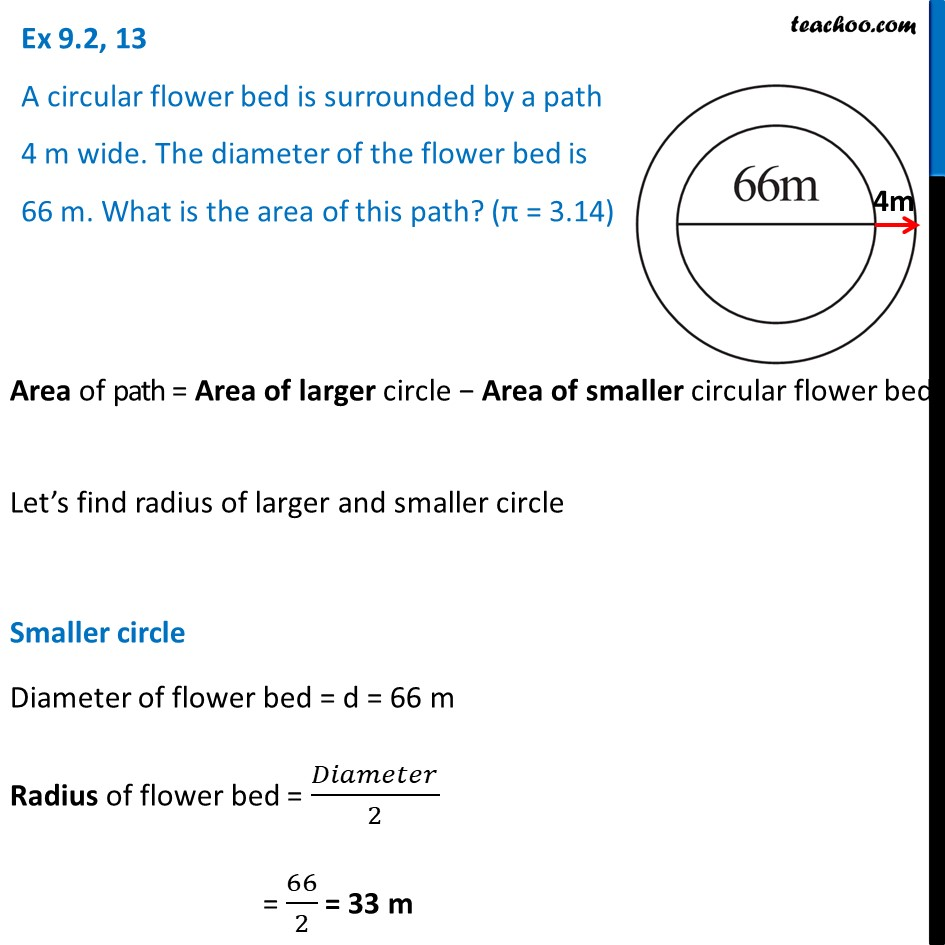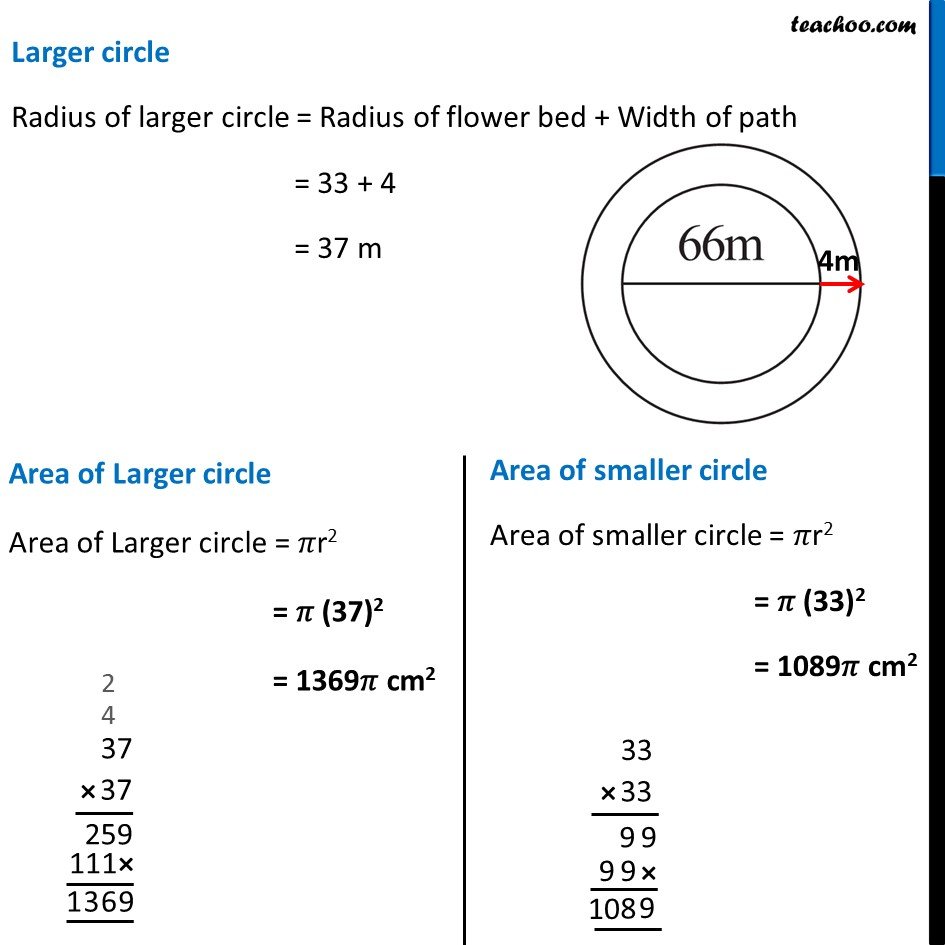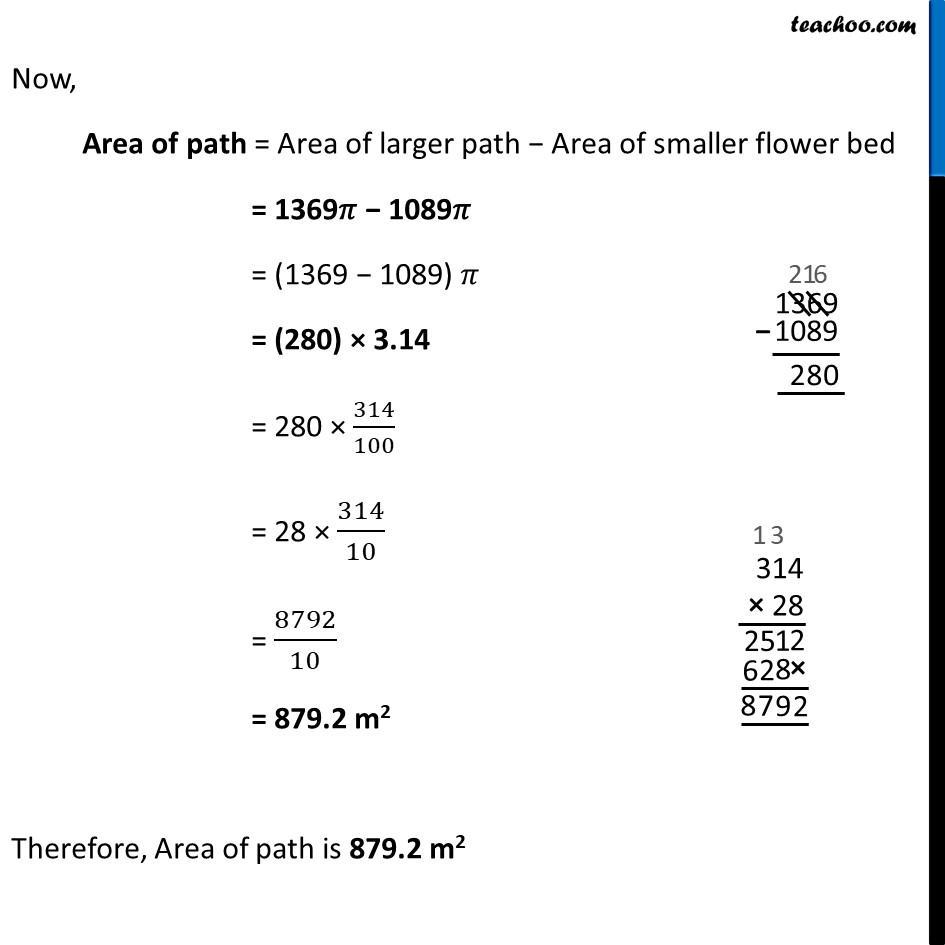Ex 9.2

Chapter 9 Class 7 Perimeter and Area
Serial order wiseLearn in your speed, with individual attention - Teachoo Maths 1-on-1 Class

### Transcript

Ex 9.2, 13 A circular flower bed is surrounded by a path 4 m wide. The diameter of the flower bed is 66 m. What is the area of this path? (π = 3.14) Area of path = Area of larger circle − Area of smaller circular flower bed Let’s find radius of larger and smaller circle Smaller circle Diameter of flower bed = d = 66 m Radius of flower bed = 𝐷𝑖𝑎𝑚𝑒𝑡𝑒𝑟/2 = 66/2 = 33 m Larger circle Radius of larger circle = Radius of flower bed + Width of path = 33 + 4 = 37 m Area of Larger circle Area of Larger circle = 𝜋r2 = 𝜋 (37)2 = 1369𝜋 cm2 Area of smaller circle Area of smaller circle = 𝜋r2 = 𝜋 (33)2 = 1089𝜋 cm2 Now, Area of path = Area of larger path − Area of smaller flower bed = 1369𝜋 − 1089𝜋 = (1369 − 1089) 𝜋 = (280) × 3.14 = 280 × 314/100 = 28 × 314/10 = 8792/10 = 879.2 m2 Therefore, Area of path is 879.2 m2